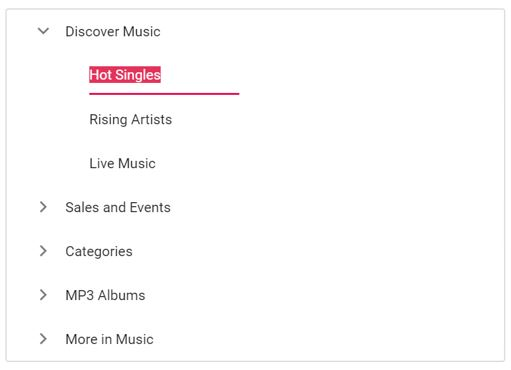# Node Editing

25 Feb 202213 minutes to read

The TreeView allows you to edit nodes by setting the allowEditing property to true.
To directly edit the nodes in place, double click the TreeView node or select the node and press F2 key.
When editing is completed by focus out or by pressing the Enter key, the modified node’s text saves automatically. If you do not want to save the modified node’s text in TreeView node, press Escape key. It does not save the edited text to the TreeView node.

• Node editing can also be performed programmatically by using the `beginEdit` method. On passing the node ID or element through this method, the edit textbox will be created for the particular node thus allowing us to edit it.

• If you need to validate or prevent editing, the `nodeEditing` event can be used which is triggered before the TreeView node is renamed. On successfully renaming a node the `nodeEdited` event will be triggered.

In the following example, the first level node’s text cannot be changed, but all other level nodes’ text can be changed.

``````@Html.EJS().TreeView("treedata").AllowEditing(true).Fields(field=>
field.Selected("is_selected").Id("id").ParentID("pid").Text("name").HasChildren("hasChild").Expanded("expanded")
.DataSource(ViewBag.dataSource)).Render()``````
``````public IActionResult NodeEdit()
{
List<object> treedata = new List<object>();
{
id = 1,
name = "Discover Music",
hasChild = true,
expanded = true
});
{
id = 2,
pid = 1,
name = "Hot Singles",

});
{
id = 3,
pid = 1,
name = "Rising Artists"
});

{
id = 4,
pid = 1,
name = "Live Music"
});
{
id = 5,
hasChild = true,
name = "Sales and Events",

});
{
id = 6,
pid=5,
name = "100 Albums - \$5 Each",
});
{
id = 7,
pid = 5,
name = "Hip-Hop and R&B Sale"
});
{
id = 8,
pid = 5,
name = "CD Deals"
});
{
id = 10,
hasChild = true,
name = "Categories"
});
{
id = 11,
pid=10,
name = "Bestselling Albums",

});
{
id = 12,
pid = 10,
name = "New Releases"
});
{
id = 13,
pid = 10,
name = "Bestselling Songs"
});
{
id = 14,
hasChild = true,
name = "MP3 Albums"
});
{
id = 15,
pid = 14,
name = "Rock"

});
{
id = 16,
name = "Gospel",
pid = 14,

});
{
id = 17,
pid = 14,
name = "Latin Music"

});
{
id = 18,
pid = 14,
name = "Jazz"

});
{
id = 19,
hasChild = true,
name = "More in Music"

});
{
id = 20,
pid = 19,
});
{
id = 21,
name = "Redeem a Gift Card",
pid = 19
});
{
id = 22,
pid = 19,
name = "Band T-Shirts"

});

ViewBag.dataSource = treedata;
return View();
}``````

Output be like the below.